An internal metric on a two-dimensional manifoldwhich meets some convexity condition. More exactly, letandbe two shortest lines issuing from some point; letandbe points on these lines; let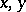be the distances from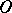toand, respectively; letbe the distance betweenand; and let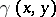be the angle opposite to the sidein the plane triangle with sides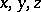. The convexity condition of the metric (at the point) is thatis a non-increasing function (i.e.if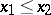,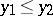) on any pair of intervals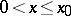,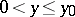such that the pointsand, which correspond to two arbitrary values in these intervals, can be connected by a shortest line. An internal metric is a convex metric if and only if it is a metric of non-negative curvature. The metric of a convex surface is a convex metric. Conversely, any two-dimensional manifold with a convex metric can be realized as a convex surface (Aleksandrov's theorem).